Latest Banking jobs   »   IBPS quant

# Quantitative Aptitude Quiz For IBPS PO Prelims 2023 -06th August

Directions (1-5): Two equations I and II are given below in each question. You have to solve these equations and give answer if
(a) if x<y
(b) if x> y
(c) if x≤ y
(d) if x≥ y
(e) if x= y or no relation can be established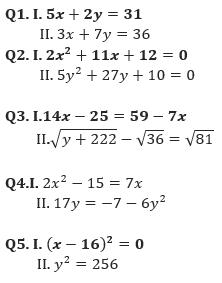Q6. Speed of boat to cover a distance in upstream is 40% of the boat to cover same distance in downstream. Find the speed of current if time taken to cover 280 km in upstream is 7 hours. (in km/hr)
(a) 25
(b) 30
(c) 20
(d) 35
(e) 40

Q7. In how many different ways the letter of words ‘MUBARAK’ be arranged so that both A’s never comes together?
(a) 1600
(b) 1760
(c) 1800
(d) 1540
(e) 1840

Q8. Ayush and Akshay invested in the ratio 1/3 :2/5 in a scheme for a time period which is in the ratio 1/5:2/3. If the profit of Ayush is Rs. 550 then find difference in profit of Akshay & Ayush?
(a) Rs. 1350
(b) Rs.1600
(c) Rs. 1450
(d) Rs. 1650
(e) Rs. 1550

Q9. Simple interest for a period of two years is Rs. 2400. If difference of CI and SI for same period is Rs. 300. Then find the rate of interest. [Amount given in each case is same & rate is also same].
(a) 12.5%
(b) 305
(c) 35%
(d) 20%
(e) 25%

Q10. The curved surface area of cylinder is 616 cm² and radius of cylinder is half the height of cylinder. Find the area of rectangle if length & breadth of rectangle is equal to height & radius of cylinder?
(a) 98 cm²
(b) None of these
(c) 256 cm²
(d) 196 cm²
(e) 144 cm²

Solutions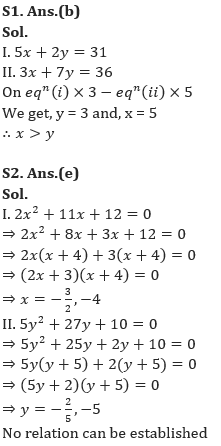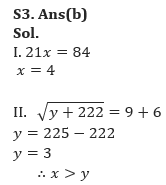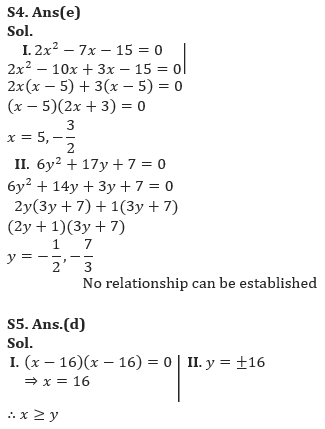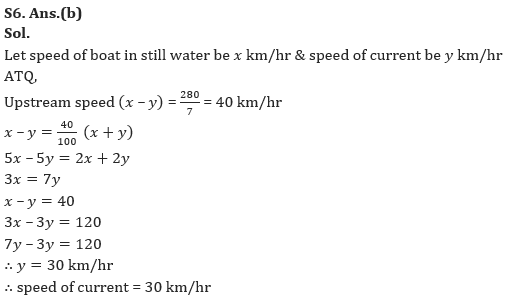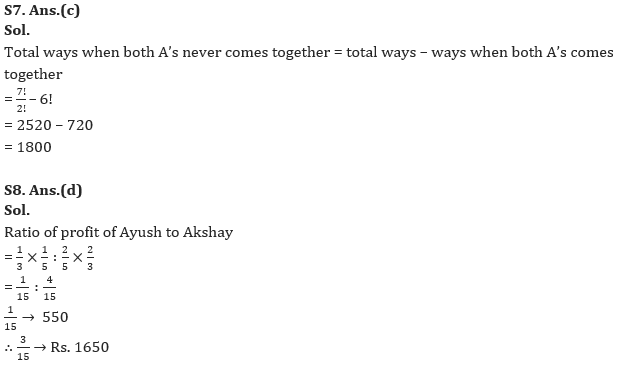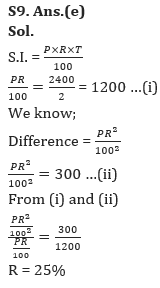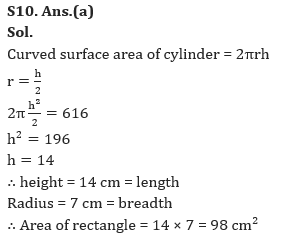## FAQs

### When will the IBPS PO prelims 2023 be conducted?

IBPS PO Prelims will be conducted on 23, 30 September, and 1 October 2023.

#### Congratulations!Union Budget 2023-24: Free PDF